## RD Sharma Class 9 Solutions Chapter 6 Factorisation of Polynomials Ex 6.4

These Solutions are part of RD Sharma Class 9 Solutions. Here we have given RD Sharma Class 9 Solutions Chapter 6 Factorisation of Polynomials Ex 6.4

Other Exercises

In each of the following, use factor Theorem to find whether polynomial g(x) is a factor of polynomial f(x) or, not: (1-7)
Question 1.
f(x) = x3 – 6x2 + 11x – 6; g(x) = x – 3
Solution:
We know that if g(x) is a factor of p(x),
then the remainder will be zero. Now,
f(x) = x3 – 6x2 + 11x – 6; g(x) = x -3
Let x – 3 = 0, then x = 3
∴ Remainder = f(3)
= (3)3 – 6(3)2 +11 x 3 – 6
= 27-54 + 33 -6
= 60 – 60 – 0
∵  Remainder is zero,
∴ x – 3 is a factor of f(x)

Question 2.
f(x) = 3X4 + 17x3 + 9x2 – 7x – 10; g(x) = x + 5
Solution:
f(x) = 3x4 + 17X3 + 9x2 – 7x – 10; g(x) = x + 5
Let x + 5 = 0, then x = -5
∴  Remainder = f(-5) = 3(-5)4 + 17(-5)3 + 9(-5)2 – 7(-5) – 10
= 3 x 625 + 17 x (-125) + 9 x (25) – 7 x (-5) – 10
= 1875 -2125 + 225 + 35 – 10
= 2135 – 2135 = 0
∵  Remainder = 0
∴ (x + 5) is a factor of f(x)

Question 3.
f(x) = x5 + 3x4 – x3 – 3x2 + 5x + 15, g(x) = x + 3
Solution:
f(x) = x5 + 3X4 – x3 – 3x2 + 5x + 15, g(x) = x + 3
Let x + 3 = 0, then x = -3
∴ Remainder = f(-3)
= (-3)5 + 3(-3)4 – (-3)3 – 3(-3)2 + 5(-3) + 15
= -243 + 3 x 81 -(-27)-3 x 9 + 5(-3) + 15
= -243 +243 + 27-27- 15 + 15
= 285 – 285 = 0
∵  Remainder = 0
∴  (x + 3) is a factor of f(x)

Question 4.
f(x) = x3 – 6x2 – 19x + 84, g(x) = x – 7
Solution:
f(x) = x3 – 6x2 – 19x + 84, g(x) = x – 7
Let x – 7 = 0, then x = 7
∴  Remainder = f(7)
= (7)3 – 6(7)2 – 19 x 7 + 84
= 343 – 294 – 133 + 84
= 343 + 84 – 294 – 133
= 427 – 427 = 0
∴  Remainder = 0
∴ (x – 7) is a factor of f(x)

Question 5.
f(x) = 3x3  + x2 – 20x + 12, g(x) = 3x – 2
Solution: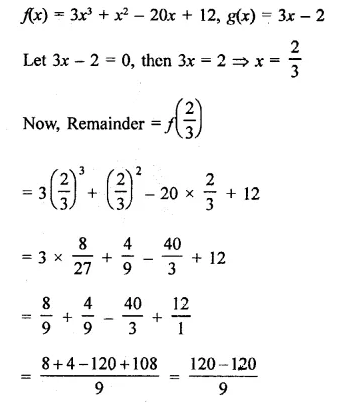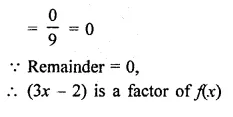Question 6.
f(x) = 2x3 – 9x2 + x + 12, g(x) = 3 – 2x
Solution:
f(x) = 2x3 – 9x2 + x + 12, g(x) = 3 – 2x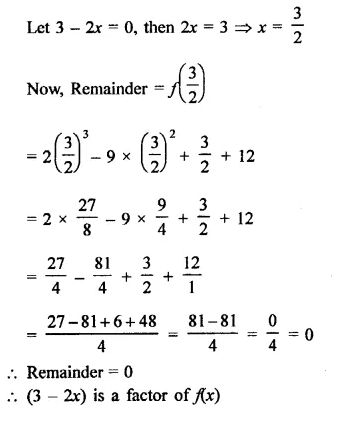Question 7.
f(x) = x3 – 6x2 + 11x – 6, g(x) = x2 – 3x + 2
Solution:
g(x) = x2 – 3x + 2
= x2 – x – 2x + 2
= x(x – 1) – 2(x – 1)
= (x – 1) (x – 2)
If x – 1 = 0, then x = 1
‍∴ f(1) = (1)3 – 6(1)2 + 11(1) – 6
= 1-6+11-6= 12- 12 = 0
‍∴ Remainder is zero
‍∴ x – 1 is a factor of f(x)
and if x – 2 = 0, then x = 2
∴ f(2) = (2)3 – 6(2)2 + 11(2)-6
= 8 – 24 + 22 – 6 = 30 – 30 = 0
‍∴ Remainder = 0
‍∴ x – 2 is also a factor of f(x)

Question 8.
Show that (x – 2), (x + 3) and (x – 4) are factors of x3 – 3x2 – 10x + 24.
Solution:
f(x) = x3 – 3x2 – 10x + 24
Let x – 2 = 0, then x = 2
Now f(2) = (2)3 – 3(2)2 – 10 x 2 + 24
= 8 – 12 – 20 + 24 = 32 – 32 = 0
‍∴ Remainder = 0
‍∴ (x – 2) is the factor of f(x)
If x + 3 = 0, then x = -3
Now, f(-3) = (-3)3 – 3(-3)2 – 10 (-3) + 24
= -27 -27 + 30 + 24
= -54 + 54 = 0
∴ Remainder = 0
∴ (x + 3) is a factor of f(x)
If x – 4 = 0, then x = 4
Now f(4) = (4)3 – 3(4)2 – 10 x 4 + 24 = 64-48 -40 + 24
= 88 – 88 = 0
∴ Remainder = 0
∴ (x – 4) is a factor of (x)
Hence (x – 2), (x + 3) and (x – 4) are the factors of f(x)

Question 9.
Show that (x + 4), (x – 3) and (x – 7) are factors of x3 – 6x2 – 19x + 84.
Solution:
Let f(x) = x3 – 6x2 – 19x + 84
If x + 4 = 0, then x = -4
Now, f(-4) = (-4)3 – 6(-4)2 – 19(-4) + 84
= -64 – 96 + 76 + 84
= 160 – 160 = 0
∴ Remainder = 0
∴ (x + 4) is a factor of f(x)
If x – 3 = 0, then x = 3
Now, f(3) = (3)3 – 6(3)2 – 19 x 3 + 84
= 27 – 54 – 57 + 84
= 111 -111=0
∴ Remainder = 0
∴ (x – 3) is a factor of f(x)
and if x – 7 = 0, then x = 7
Now, f(7) = (7)3 – 6(7)2 – 19 x 7 + 84
= 343 – 294 – 133 + 84
= 427 – 427 = 0
∴ Remainder = 0
∴ (x – 7) is also a factor of f(x)
Hence (x + 4), (x – 3), (x – 7) are the factors of f(x)

Question 10.
For what value of a (x – 5) is a factor of x3 – 3x2 + ax – 10?
Solution:
f(x) = x3 – 3x2 + ax – 10
Let x – 5 = 0, then x = 5
Now, f(5) = (5)3 – 3(5)2 + a x 5 – 10
= 125 – 75 + 5a – 10
= 125 – 85 + 5a = 40 + 5a
∴ (x – 5) is a factor of fix)
∴ Remainder = 0
⇒  40 + 5a = 0 ⇒  5a = -40
⇒ a = $$\frac { -40 }{ 5 }$$= -8
Hence a = -8

Question 11.
Find the value of a such that (x – 4) is a factor of 5x3 – 7x2 – ax – 28.
Solution:
Let f(x)  5x3 – 7x2 – ax – 28
and Let x – 4 = 0, then x = 4
Now, f(4) = 5(4)3 – 7(4)2 – a x 4 – 28
= 5 x 64 – 7 x 16 – 4a – 28
= 320 – 112 – 4a – 28
= 320 – 140 – 4a
= 180 – 4a
∴ (x – 4) is a factor of f(x)
∴ Remainder = 0
⇒  180 -4a = 0
⇒  4a = 180
⇒  a = $$\frac { 180 }{ 4 }$$ =  45
∴  a = 45

Question 12.
Find the value of a, if x + 2 is a factor of 4x4 + 2x3 – 3x2 + 8x + 5a.
Solution:
Let f(x) = 4x4 + 2x3 – 3x2 + 8x + 5a
and Let x + 2 = 0, then x = -2
Now, f(-2) = 4(-2)4 + 2(-2)3 – 3(-2)2 + 8 x ( 2) + 5a
= 4 x 16 + 2(-8) – 3(4) + 8 (-2) + 5a
= 64- 16- 12- 16 +5a
= 64 – 44 + 5a
= 20 + 5a
∴  (x + 2) is a factor of fix)
∴  Remainder = 0
⇒  20 + 5a = 0 ⇒  5a = -20
⇒  a =$$\frac { -20 }{ 5 }$$  = -4
∴ a = -4

Question 13.
Find the value of k if x – 3 is a factor of k2x3 – kx2 + 3kx – k.
Solution:
Let f(x) = k2x3 – kx2 + 3kx – k
and Let x – 3 = 0, then x = 3
Now,f(3) = k2(3)3 – k(3)2 + 3k(3) – k
= 27k2 – 9k + 9k-k
= 27k2-k
∴ x – 3 is a factor of fix)
∴ Remainder = 0
∴ 27k2 – k = 0
⇒ k(27k – 1) = 0 Either k = 0
or 21k – 1 = 0
⇒ 21k = 1
∴  k= $$\frac { 1 }{ 27 }$$
∴  k = 0,$$\frac { 1 }{ 27 }$$

Question 14.
Find the values of a and b, if x2 – 4 is a factor of ax4 + 2x3 – 3x2 + bx – 4.
Solution:
f(x) = ax4 + 2x3 – 3x2 + bx – 4
Factors of x2 – 4 = (x)2 – (2)2
= (x + 2) (x – 2)
If x + 2 = 0, then x = -2
Now, f(-2) = a(-2)4 + 2(-2)3 – 3(-2)2 + b(-2) – 4
16a- 16 – 12-26-4
= 16a -2b-32
∵ x + 2 is a factor of f(x)
∴ Remainder = 0
⇒  16a – 2b – 32 = 0
⇒ 8a – b – 16 = 0
⇒ 8a – b = 16         …(i)
Again x – 2 = 0, then x = 2
Now f(2) = a x (2)4 + 2(2)3 – 3(2)2 + b x 2-4
= 16a + 16- 12 + 26-4
= 16a + 2b
∵  x – 2 is a factor of f(x)
∴ Remainder = 0
⇒  16a + 2b = 0
⇒ 8a + b= 0                             …(ii)
⇒ 16a = 16
⇒ a = $$\frac { 16 }{ 16 }$$ = 1
From (ii) 8 x 1 + b = 0
⇒ 8 + b = 0
⇒  b = – 8
∴ a = 1, b = -8

Question 15.
Find α and β, if x + 1 and x + 2 are factors of x3 + 3x2 – 2αx +β.
Solution:
Let f(x) = x3 + 3x2 – 2αx + β
and Let x + 1 = 0 then x = -1
Now,f(-1) = (1)3 + 3(-1)2 – 2α (-1) +β
= -1 + 3 + 2α + β
= 2 + 2α + β
∵  x + 1 is a factor of f(x)
∴  Remainder = 0
∴ 2 + 2α + β = 0
⇒  2α + β = -2                    …(i)
Again, let x + 2 = 0, then x = -2
Now, f(-2) = (-2)3 + 3(-2)2 – 2α(-2) + β
= -8 + 12 + 4α+ β
= 4 + 4α+ β
∵ x + 2 is a factor of(x)
∴ Remainder = 0
∴ 4+ 4α + β = 0
⇒  4α + β = -4 …(ii)
Subtracting (i) from (ii),
2α = -2
⇒  α = $$\frac { -2 }{ 2 }$$ = -1
From (ii), 4(-1) + β = -4
-4 + β= -4
⇒  β =-4+ 4 = 0
∴  α = -1, β = 0

Question 16.
If x – 2 is a factor of each of the following two polynomials, find the values of a in each case:
(i) x3 – 2ax2 + ax – 1
(ii) x5 – 3x4 – ax3 + 3ax2 + 2ax + 4
Solution:
(i) Let f(x) = x3 – 2ax2 + ax – 1 and g(x) = x – 2
and let x – 2 = 0, then x = 2
∴ x – 2 is its factor
∴ Remainder = 0
f(2) = (2)3 – 2a x (2)2 + a x 2 – 1
= 8-8a+ 2a-1 = 7-6a
∴ 7 – 6a = 0
⇒  6a = 7
⇒ a = $$\frac { 7 }{ 6 }$$
∴ a =  $$\frac { 7 }{ 6 }$$
(ii) Let f(x) = x5 – 3x4 – ax3 + 3 ax2 + 2ax + 4 and g(x) = x – 2
Let x – 2 = 0, then x=2
∴ f(2) = (2)5 – 3(2)4 – a(23) + 3a (2)2 + 2a x 2 + 4
= 32 – 48 – 8a + 12a + 4a + 4
= -12 + 8a
∴ Remainder = 0
∴ -12 + 8a = 0
⇒ 8a= 12
⇒ a = $$\frac { 12 }{ 8 }$$ = $$\frac { 3 }{ 2 }$$
∴ Hence a = $$\frac { 3 }{ 2 }$$

Question 17.
In each of the following two polynomials, find the values of a, if x – a is a factor:
(i) x6 – ax5 + x4-ax3 + 3x-a + 2
(ii) x5 – a2x3 + 2x + a + 1
Solution:
(i) Let f(x) = x– ax5+x4-ax3 + 3x-a + 2 and g(x) = x – a
∴ x – a is a factor
∴ x – a = 0
⇒ x = a
Now f(a) = a6-a x a5 + a4-a x a3 + 3a – a + 2
= a6-a6 + a4-a4 + 2a + 2
= 2a + 2
∴ x + a is a factor of p(x)
∴ Remainder = 0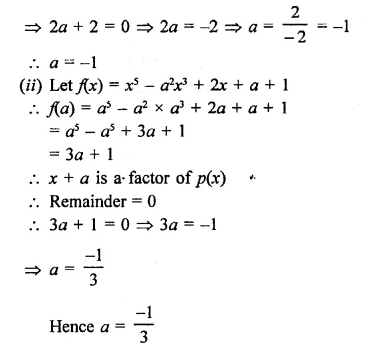Question 18.
In each of the following, two polynomials, find the value of a, if x + a is a factor.
(i)  x3 + ax2 – 2x + a + 4
(ii) x4 – a2r + 3x – a
Solution: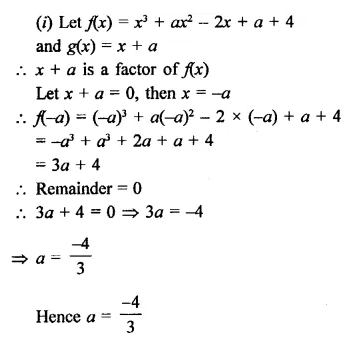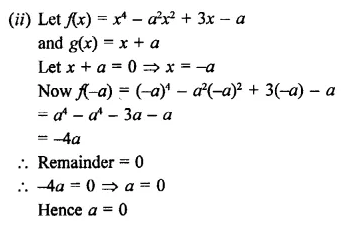Question 19.
Find the values of p and q so that x4 + px3 + 2x2 – 3x + q is divisible by (x2 – 1).
Solution: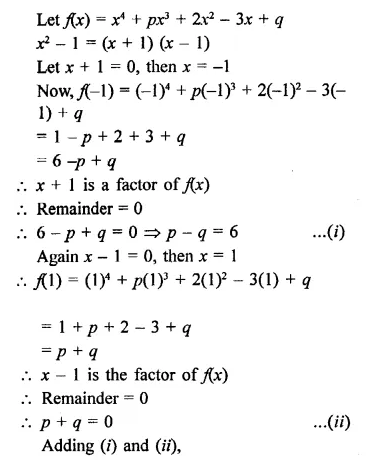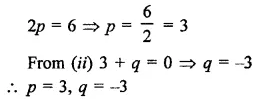Question 20.
Find the values of a and b so that (x + 1) and (x – 1) are factors of x4 + ax3 3x2 + 2x + b.
Solution: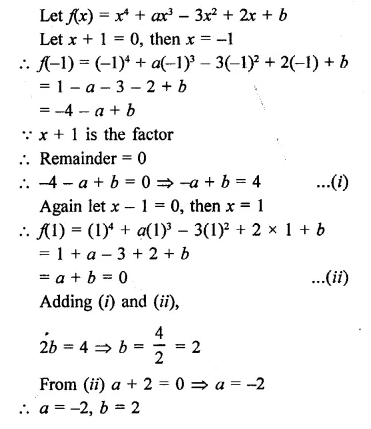Question 21.
If x3 + ax2 – bx + 10 is divisible by x2 – 3x + 2, find the values of a and b.
Solution:Question 22.
If both x + 1 and x – 1 are factors of ax3 + x2 – 2x + b, find the values of a and b.
Solution:Question 23.
What must be added to x3 – 3x2 – 12x + 19 so that the result is exactly divisibly by x2 + x – 6?
Solution: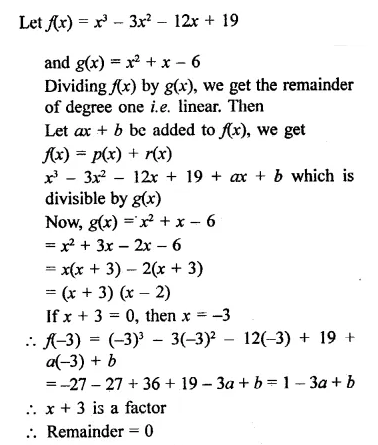Question 24.
What must be subtracted from x3 – 6x2 – 15x + 80-so that the result is exactly divisible by x2 + x – 12?
Solution: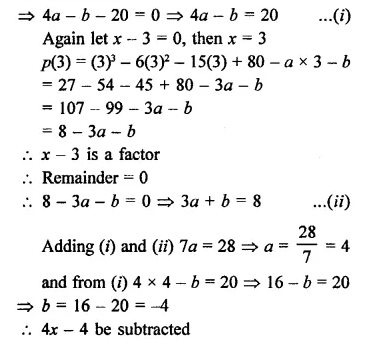Question 25.
What must be added to 3x3 + x2 – 22x + 9 so that the result is exactly divisible by 3x2 + 7x – 6?
Solution: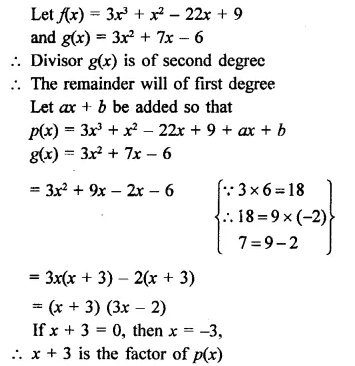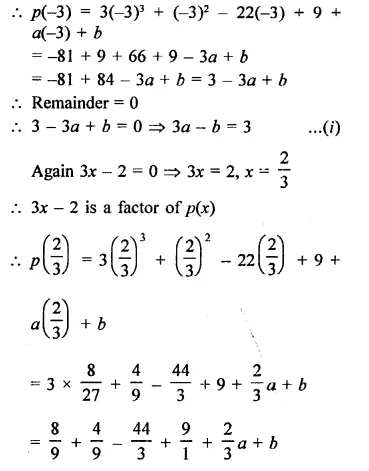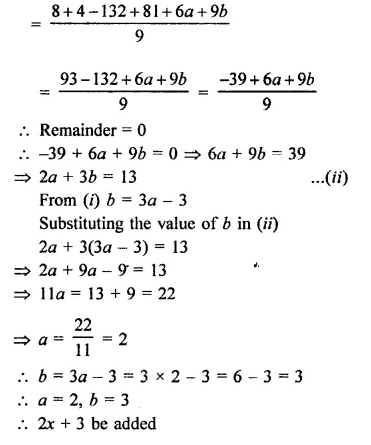Hope given RD Sharma Class 9 Solutions Chapter 6 Factorisation of Polynomials Ex 6.4 are helpful to complete your math homework.

If you have any doubts, please comment below. Learn Insta try to provide online math tutoring for you.